# C PROGRAMMING p-3.pptx

Assistant Professor em D.K.M college for women
27 de May de 2023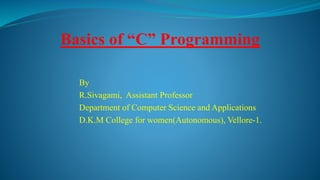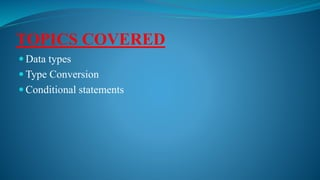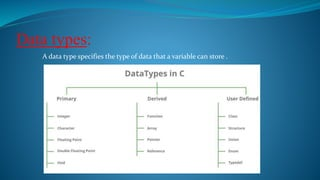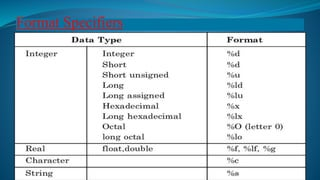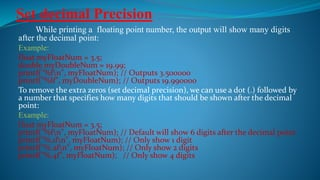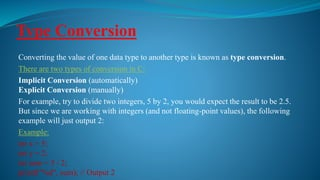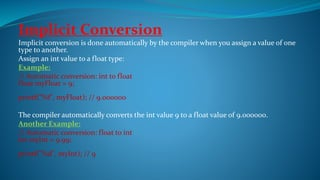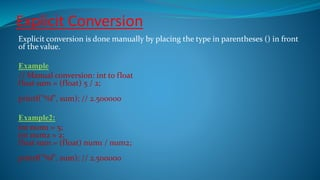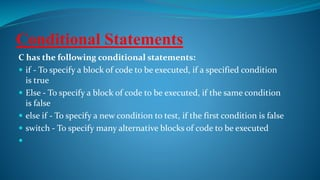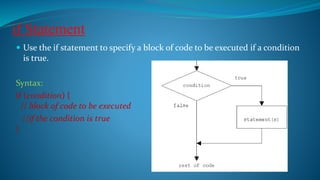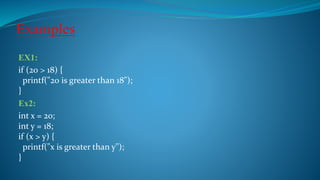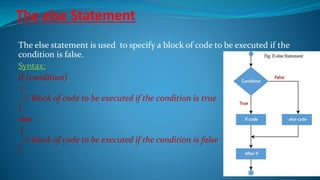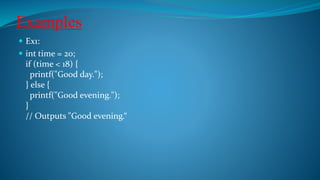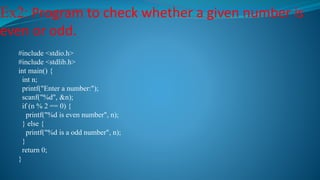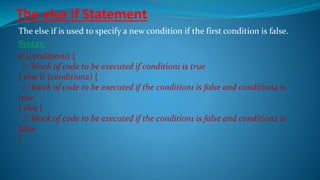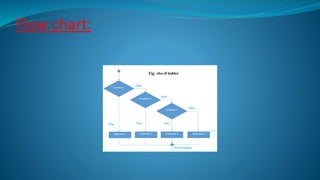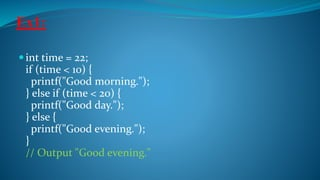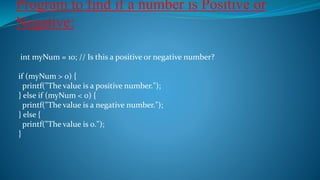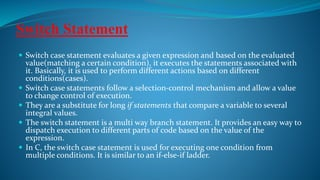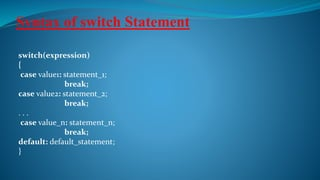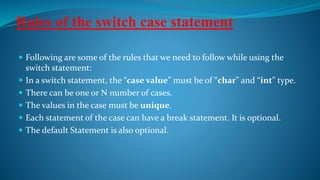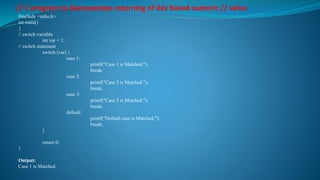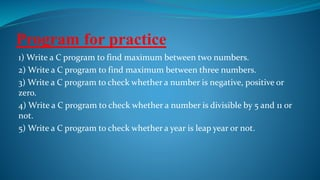1 de 24

### C PROGRAMMING p-3.pptx

• 1. Basics of “C” Programming By R.Sivagami, Assistant Professor Department of Computer Science and Applications D.K.M College for women(Autonomous), Vellore-1.
• 2. TOPICS COVERED  Data types  Type Conversion  Conditional statements
• 3. Data types: A data type specifies the type of data that a variable can store .
• 5. Set decimal Precision While printing a floating point number, the output will show many digits after the decimal point: Example: float myFloatNum = 3.5; double myDoubleNum = 19.99; printf("%fn", myFloatNum); // Outputs 3.500000 printf("%lf", myDoubleNum); // Outputs 19.990000 To remove the extra zeros (set decimal precision), we can use a dot (.) followed by a number that specifies how many digits that should be shown after the decimal point: Example: float myFloatNum = 3.5; printf("%fn", myFloatNum); // Default will show 6 digits after the decimal point printf("%.1fn", myFloatNum); // Only show 1 digit printf("%.2fn", myFloatNum); // Only show 2 digits printf("%.4f", myFloatNum); // Only show 4 digits
• 6. Type Conversion Converting the value of one data type to another type is known as type conversion. There are two types of conversion in C: Implicit Conversion (automatically) Explicit Conversion (manually) For example, try to divide two integers, 5 by 2, you would expect the result to be 2.5. But since we are working with integers (and not floating-point values), the following example will just output 2: Example: int x = 5; int y = 2; int sum = 5 / 2; printf("%d", sum); // Output 2
• 7. Implicit Conversion Implicit conversion is done automatically by the compiler when you assign a value of one type to another. Assign an int value to a float type: Example: // Automatic conversion: int to float float myFloat = 9; printf("%f", myFloat); // 9.000000 The compiler automatically converts the int value 9 to a float value of 9.000000. Another Example: // Automatic conversion: float to int int myInt = 9.99; printf("%d", myInt); // 9
• 8. Explicit Conversion Explicit conversion is done manually by placing the type in parentheses () in front of the value. Example // Manual conversion: int to float float sum = (float) 5 / 2; printf("%f", sum); // 2.500000 Example2: int num1 = 5; int num2 = 2; float sum = (float) num1 / num2; printf("%f", sum); // 2.500000
• 9. Conditional Statements C has the following conditional statements:  if - To specify a block of code to be executed, if a specified condition is true  Else - To specify a block of code to be executed, if the same condition is false  else if - To specify a new condition to test, if the first condition is false  switch - To specify many alternative blocks of code to be executed 
• 10. if Statement  Use the if statement to specify a block of code to be executed if a condition is true. Syntax: if (condition) { // block of code to be executed //if the condition is true }
• 11. Examples EX1: if (20 > 18) { printf("20 is greater than 18"); } Ex2: int x = 20; int y = 18; if (x > y) { printf("x is greater than y"); }
• 12. The else Statement The else statement is used to specify a block of code to be executed if the condition is false. Syntax: if (condition) { // block of code to be executed if the condition is true } else { // block of code to be executed if the condition is false }
• 13. Examples  Ex1:  int time = 20; if (time < 18) { printf("Good day."); } else { printf("Good evening."); } // Outputs "Good evening.“
• 14. Ex2: Program to check whether a given number is even or odd. #include <stdio.h> #include <stdlib.h> int main() { int n; printf("Enter a number:"); scanf("%d", &n); if (n % 2 == 0) { printf("%d is even number", n); } else { printf("%d is a odd number", n); } return 0; }
• 15. The else if Statement The else if is used to specify a new condition if the first condition is false. Syntax: if (condition1) { // block of code to be executed if condition1 is true } else if (condition2) { // block of code to be executed if the condition1 is false and condition2 is true } else { // block of code to be executed if the condition1 is false and condition2 is false }
• 17. Ex1: int time = 22; if (time < 10) { printf("Good morning."); } else if (time < 20) { printf("Good day."); } else { printf("Good evening."); } // Output "Good evening."
• 18. Program to find if a number is Positive or Negative: int myNum = 10; // Is this a positive or negative number? if (myNum > 0) { printf("The value is a positive number."); } else if (myNum < 0) { printf("The value is a negative number."); } else { printf("The value is 0."); }
• 19. Switch Statement  Switch case statement evaluates a given expression and based on the evaluated value(matching a certain condition), it executes the statements associated with it. Basically, it is used to perform different actions based on different conditions(cases).  Switch case statements follow a selection-control mechanism and allow a value to change control of execution.  They are a substitute for long if statements that compare a variable to several integral values.  The switch statement is a multi way branch statement. It provides an easy way to dispatch execution to different parts of code based on the value of the expression.  In C, the switch case statement is used for executing one condition from multiple conditions. It is similar to an if-else-if ladder.
• 20. Syntax of switch Statement switch(expression) { case value1: statement_1; break; case value2: statement_2; break; . . . case value_n: statement_n; break; default: default_statement; }
• 21. Rules of the switch case statement  Following are some of the rules that we need to follow while using the switch statement:  In a switch statement, the “case value” must be of “char” and “int” type.  There can be one or N number of cases.  The values in the case must be unique.  Each statement of the case can have a break statement. It is optional.  The default Statement is also optional.
• 22. // C program to Demonstrate returning of day based numeric // value #include <stdio.h> int main() { // switch variable int var = 1; // switch statement switch (var) { case 1: printf("Case 1 is Matched."); break; case 2: printf("Case 2 is Matched."); break; case 3: printf("Case 3 is Matched."); break; default: printf("Default case is Matched."); break; } return 0; } Output: Case 1 is Matched.
• 23. Program for practice 1) Write a C program to find maximum between two numbers. 2) Write a C program to find maximum between three numbers. 3) Write a C program to check whether a number is negative, positive or zero. 4) Write a C program to check whether a number is divisible by 5 and 11 or not. 5) Write a C program to check whether a year is leap year or not.